## Introduction

Lipid membranes mimic red blood cells (RBC) albeit in an oversimplified way. Besides the lipid bilayer, RBC possess a spectrin mesh for extra shear-resistance. Malaria, acanthocytosis and echynocytosis are examples of pathologies in which RBC suffer deformations that are due to defects in the spectrin network, such as dissociation in the case of malaria, which induces a permanent shape change1 or a symmetry breaking between the two leaflets of the lipid membrane in the case of acanthocytes2. The mechanics of lipid membranes were modeled using an elastic formalism borrowed from elasic sheets3,4 but so far no plastic behavior has been observed nor documented. Plasticity is often attributed to dislocation dynamics in metals5 and to volume increases in polymers6. It is, however, not clear whether plastic deformations are the result of such kinematics and flows or if they cause them. Stress condensation on ridges7 and vertices8 during the crumpling process of thin sheets is an example of when the onset of elastoplasticity is induced by volume reduction9,10. Whereas crumpling of thin sheets has emerged as an archetype of disordered systems, the aforementioned studies focused on elastic behavior, which restricts the richness of these systems. Large deformations, however, induce real-life sheets to behave plastically. Elastic crumpling is characterized by a hierarchical cascade during which large folds break into smaller folds until compaction11. At “weak” confinement, the competition between bending and stretching dominates the self-avoidance of the sheet, whereas at stronger confinement, when making a ball of radius R, for instance, the sheet's self-avoidance hinders the formation of smaller folds12. The interplay between self-avoidance and elasticity manifests itself in the Flory relationship, an important result from polymer physics, which states that the radius, R, of the crumpled configuration scales with the size of the system, L, such that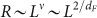, where ν is the Flory exponent and dF is the fractal dimension that measures how the sheet's size affects the mechanical compaction. This result holds for crumpling at a constant force and the identity ν = 2/dF is for maximally compacted two-dimensional surfaces and holds for different materials13,14,15,16. For elastic sheets, dF ~ 2.5. For plastically deformed macroscopic sheets this relationship holds but with a different exponent ν and dF < 2.517.

At the micro- and nanoscales, conformations of crumpled graphite oxide sheets were probed experimentally using light and X-rays scattering and the fractal dimension was found to be that of deformed elastic sheets with dF ~ 2.518,19. Membranes, made of partially polymerized diacetylenic phospholipids and cooled below the chain melting temperature, Tm, undergo a thermodynamic wrinkling transition, similar to the wrinkling of an elastic macroscopic sheet, where the vesicles abruptly fold, wrinkle, collapse and expel their payload20,21. Atomic Force Microscope (AFM) profilometry on these crumpled lipid membranes revealed that their surfaces were self-similar and behaved like elastic sheets up to the degree of polymerization after which the roughness exponent fell into a different universality class also called a glassy phase21. The mechanics that describe this phase are still lacking however. Furthermore, profilometry only probes the envelope of the surface of the wrinkled membrane and does not access the convoluted three-dimensional (3D) conformation of the membrane nor does it give sufficient information on the local mechanics of the wrinkled membrane. To probe the mechanical behaviour of these membranes we use a scattering technique. Since X-rays uncontrollably polymerise the membranes, we used neutron scattering to gain access to the local structure while measuring the fractal dimension of these self-similar structures18. The scattering intensity is proportional to the structure factor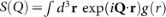, where g(r) is the pair correlation function, which is a power law for a fractal object andforandfor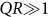22,23,24. Small Angle Neutron Scattering at the Argonne National Laboratory (Illinois) enabled us to probe length-scales from 15 to 1500 Å such that, where R is the size of the vesicle (on the order of tens of microns) and 1/Q is the observation scale. We expected that if the wrinkled membranes were self-similar and fractal, the scattered intensity would be expressed as a power-law in Q. (Data analysis is explained in the section Materials and Methods).

## Results

Figure 1 left panel presents the plot of the scattered neutron intensity versus the wavenumber for lipid tubules dispersed in water. As shown in the figure, when Q is smaller than 0.1 Å−1, the data from all samples fall on a line best fitted to the function, where dF is the fractal dimension. It is a constant and approximately equal to 3, within experimental error, which is characterestic of mass fractals25. Around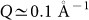, the intensity has a local peak at Q = 0.094 Å−1 after which it drops, following a power law of the form Q−4 corresponding to the Porod regime for sharp interfaces26. This partial peak corresponds to an interlayer distance of 6.68 nm for tubules in the crystalline phase where the bilayers consist of tilted chain-frozen lipids. This value agrees with previous results using X-rays27. When the tubules are heated to 45°C, they swell into vesicles with a characteristic bilayer thickness given by the peak in the intensity at Q = 0.088 Å−1 as shown in Figure 1 right panel. This peak's position, which does not depend on the degree of polymerization, corresponds to an interlayer distance, d = 7.14 nm higher than twice the lipid chain length.

We systematically varied the degree of polymerization and captured the scattering spectra from the membranes. Figure 2a shows the intensities from vesicles with low and high degrees of polymerization when the temperature was kept at 15°C. Unlike the plots in Figure 1-left panel, the slope of the intensity spectrum for Q < 0.1Å−1 shows a clear dependence on the degree of polymerization. The fractal dimension, retrieved from the slopes for different degrees of polymerization and shown in Figure 2b, remains constant at around 2.5 over a wide range of degrees of polymerization. At a critical degree of polymerization ϕc ~ 30% it drops reaching the value of 2.05 when ϕ ~ 40%. This behavior of dF is similar to the behavior of the roughness exponent for these membranes21. The surfaces for which df < 3 are clearly different from rough surface fractals (3 < dF < 4) or mass fractals (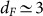), however. One wonders about the mechanism behind the drop in the value of the fractal dimension when the degree of polymerization crosses a critical value.

Step IV in Figure 3 demonstrates our new capability to heat the dispersed wrinkled vesicles beyond Tm. When crumpled vesicles that were polymerized below 30% were heated beyond Tm, they recovered their spherical shapes. Their corresponding intensities are given in the bottom curve in Figure 2c. Crumpled vesicles that are polymerized above 30% and heated beyond Tm had the same scattering intensity as the ones kept at low temperature as shown in Figure 2d. The absence of the interlayer peak and the presence of a cross-over at Q ~ 0.1 is inherent in a typical thickness with defects in the membrane. Similar cross-overs have been observed in the spectrin networks of red blood cells (RBCs) when probed with light and X-ray scatterings28. At polymerizations beyond a critical value ϕc ~ 30%, the vesicles that are heated to temperatures around 45 or 50°C do not recover their spherical shapes anymore as shown by the scattering intensity shown in Figure 2d, where the acquisition lasted for 12 hours, as well as by phase contrast microscopy. They were indeed locked in a wrinkled configuration via plastic deformation. The representative peaks observed at higher temperatures disappear and the intensity plots are similar with equal slopes equal to 2.25. The vesicles do not unwrinkle and stay trapped in a plastic state. The plots in (3-a) and (3-d) are offset vertically for clarity.

## Discussion

As mentioned above, lipid membranes are well described using continuum elasticity theory. Previous experiments on membranes and graphene sheets revealed that their behaviour is universal and scale independent – lipid membranes behave just like macroscopic elastic sheets. Given the plethora of numerical studies that use continuum mechanics to describe lipid membranes29, it is then justifiable to extend results from numerical studies on macroscopic sheets to microscopic sheets such as lipid membranes. Recent numerical simulations30, in which a thin sheet of lateral dimension L was packed and crumpled into a ball of radius R under constant force, showed that if the scaling behaviorholds, the fractal dimension depends weakly on the applied crumpling force. These simulations further showed that when the material was purely elastic, dF = 2.5 remained constant; when the material was elastoplastic however, the fractal dimension decreases from 2.5 to lower values, approaching 2, with the yield stress, σy, also decreasing. Similar trend was found in real crumpled plastic foils that were experimentally folded into balls17. We recall that the Flory exponent, ν, which relates the lateral dimension to the size of the confining space or the packed configuration through R ~ Lν, is related to the fractal dimension, for maximum packing only, via the relation ν = D/dF, where D = 2 is the dimension of the sheet14. Our observations here allow us to draw various conclusions. Figure 2b shows that the membrane has properties similar to an elastic sheet (i.e. mylar or graphene18) at low degrees of polymerisation and to that of a plastic sheet (i.e. aluminum foil17) at high degrees of polymerisation. Thus, the two distinct states we observed are related to the transition from elastic to elastoplastic behavior. Moreover, increasing the degrees of polymerization causes the membrane to behave elastoplastically and the yield stress becomes a decreasing function of the degree of polymerization, σy/E = f(ϕ), where E is the Young's modulus. Based on the analogies above and that lipid membranes have similar behaviour to macroscopic elastic sheets, we can estimate the value of the yield stress for these polymerised membranes by comparing the values of the fractal dimension for a given yield stress reported in30 to the fractal dimensions reported in this study. In30 the universality of the folding pattern was a function of the ratio of the length of the sheet to its thickness which indicates that the scale of the sheet is irrelevant as long as the ratio of the folds to the bilayer's thickness is above 100. The non-universality and the dependence of the crumpling on this ratio was attributed to plastic yielding. We find that (ϕ; σy/E) = (35%;0.05), (36.8%;0.01), (39%;0.002). While the elastoplasticity of macroscopic objects has been characterized in three dimensions only, it is possible to relate elastoplasticity to glassy behavior at the microscopic level in lipid membranes31 where defects (disclinations or dislocations) created by the polymerization cause the membrane to behave like a plastic solid32. Also and as shown before and using generalised polarisation measurements21, the wrinkling temperature depends on the degree of polymerisation. This is possibly due to a competition between the local intrinsic strain which originates from the polymer network and gives rise to a non-zero gaussian curvature and the possible configurations of the lipids. Although we submitted the vesicles to constant loading [T1T2], the wrinkling occured at a temperature that was never below 15°C. We have compared the fractal dimension values with the values of the roughness exponents and we found a slight correlation beyond the critical polymerization, which we believe is merely coincidental, but surprisingly no correlation below the critical polymerization where both exponents are constant. This could be expected since the roughness exponent measured using AFM underestimated the mechanics of the folding process during the wrinkling which gives rise to an area that is larger than the effective surface measured by the AFM. For rough surfaces generated from fracture for example, the two exponents are linearly dependent33.

We now focus on the power-law dependence of the fractal dimension on the degree of polymerisation. According to Figure 2b,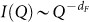and dF ~ ϕ−1/2. The dependence of the fractal dimension on ϕ means that the elastic moduli depend on ϕ. As a first approximation, we assume the Ansatz that K ~ kB, where K is the splay modulus34. I(Q) is proportional to the neutron scattering structure factor, S(Q), which is proportional to the density-density correlation function. If the Hamiltonian used to calculate the correlation function is a function only of the Lamé coefficients as in the case of a crystalline membrane or contains only a bending term, the correlation function behaves like a power-law with the exponent depending linearly on the elastic constant35. For the exponent to depend on ϕ1/2, the elastic modulus must have the same exponent within the above approximation. As shown earlier, the bilayer becomes compressed when the vesicles are cooled. This suggests that, when calculating the correlation function, we should use the Landau-Peierls Hamiltonian that describes the compression of a smectic-A that accompanies splay36. This Hamiltonian contains a compression term and is proportional to the compressibility of the layers, B(∂u/∂z)2, as well as a bending term, K(∂2u/∂x2 + ∂2u/∂y2)2. The membranes being polymerized would experience extra stretching on the outer layer like an accordion. Since the membrane is not fully polymerized, we do not need to introduce the Lamé coefficients. If we use this Hamiltonian to calculate the intensity, we find that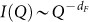, where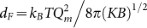. Since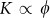, then. It is also possible that the polymer is inducing an unbinding transition of the bilyaer which causes the bilayer to compress when cooled and unbind when heated, due to bending modes in the absence of interlamellar contacts37,38,39.

Noticeably, the topology of the broken spectrin mesh during malaria infection of RBCs is somewhat similar to the random polymerization described above1. RBCs experience permanent deformations in the form of echinoytosis either in patients with liver diseases40 or in patients with Low Density Lipoprotein (LDL) deficiency2 and malaria41. These deformations were interpreted as a consequence of the dissociation of the membrane from the spectrin mesh. When these cells flow into capillaries, they experience large deformations in the form of plastic flow42. The random break-up of the spectrin mesh could create defects on the surface of the membranes1, which would cause the deformation to become plastic. Such process can be described using the newly developed model of plastic flow in glassy and amorphous materials43,44. On the other hand the contour of cancer cells was found to display a fractal behavior that would help identifying cancer cells from normal cells45,46.

## Methods

We used polymerizable lipids [1,2-bis(10,12-tricosadiynoyl)-sn-glycero-3-phosphocholine] purchased from Avanti Lipids as powder and stored at −20°C. When spherical vesicles made of these lipids are cooled below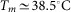, they undergo a first-order type of shape transformation from spherical vesicles to helical ribbons and tubules27. In two-dimensions monolayers of these lipids form spirals below Tm and a target pattern above Tm21,47. The transformation from tubules/ribbons to vesicles when the temperature is increased is also caused by the relaxation of the chiral torque due to thermal fluctuations48. We prepared the vesicles using the hydration method: A lipid film from a stock solution of 6 mg/ml in chloroform was spread on a concave surface of a custom-made teflon container and evaporated over night under a stream of nitrogen. We hydrated the lipid film with deuterated methanol:water (Aldrich, Inc.) [75:25 (v:v)] to a lipid concentration of 3 mg/ml and kept it in an oven at 50°C for several hours to allow the vesicles to swell. This ensured that the vesicles obtained were Giant Unilamellar Vesicles (GUVs). We heated the vesicles to various temperatures above Tm and the results were not affected. We used the hydration method in conjunction with the water/alcohol mixing to produce GUV preferably to the electroformation method because we wanted to avoid any electrostatic effects that migh affect the membranes' properties. Since the lipid molecules were not deuterated, the contrast variation stemmed from the difference between the deuterated solvent and the lipid bilayer. The contrast in the scattering will be between the bilayer and the deuterated water solution. We cooled the vesicles below Tm at a rate of 1°C/hour while we kept them in the dark. We polymerized the lipid molecules by exposing the tubules that were kept at 15°C to a UV lamp set at 254 nm and with an intensity of 4 μW/cm2. We measured the degree of polymerization as the area under the absorption curve of the polymer using a UV/Vis spectrometer (Genesys 10S)49. For our scattering experiments, we prepared various samples each of which corresponded to a separate experiment or step as illustrated in Figure 3.

The control samples, Step I in Figure 3, included tubules maintained at 15°C ± 0.1°C throughout the scattering experiment in 1-mm-thick quartz cells. We prepared another set of samples by reheating the polymerized vesicles to 45°C and keeping them in this state for several hours before letting them cool to 15°C when they wrinkled before we started the scattering experiments (Step III). The last set of samples were similar to the second set except that we heated them to 45°C and performed the scattering experiments at this temperature. This is shown as Step IV in Figure 3. When changing temperatures, we allowed several hours for the vesicles to equilibrate at the prescribed temperature before we started the data acquisition at the new temperature. The neutron scattering and data acquisition ran for 12 hours continuously.

### Data analysis and background correction

The sample's cross section is measured by subtracting the empty cell intensity from the total intensity. The transmission are computed with respect to an empty beam by using a beam stopper and record electronic background that needs to be subtracted. As seen from the intensity at high Q (≥0.4) the incoherent scattering is rather flat and this is due to the solid angle correction from geometric distortion. Although the diminution in the transmission through heavy water is around 3%, which is less than the diminution at high Q due to geometric distortion, this has been corrected with empty cells containing D2O. Because we used thin quartz cells that are 500 μm thick, multiple scattering was minimized. Substraction of incoherent background which is visible mostly at high Q is performed by empty cell substraction as well as solvent without structure subtraction. At high Q we plot I(Q)Q−4 versus Q. The slope gives the value of incoherent background which is also reduced by using as little hydrogen atoms as possible. Using a deuterated solvent reduces greatly the incoherent background because of the large differences between the cross section and scattering densities of hydrogen and deuterium atoms. As seen from all scattering plots, the incoherent scattering (at high Q) is almost flat.